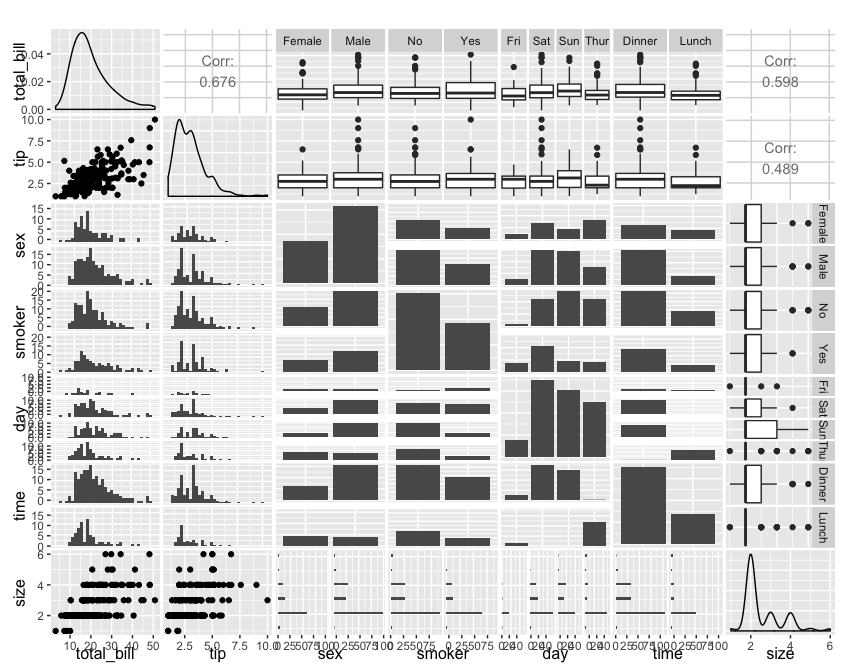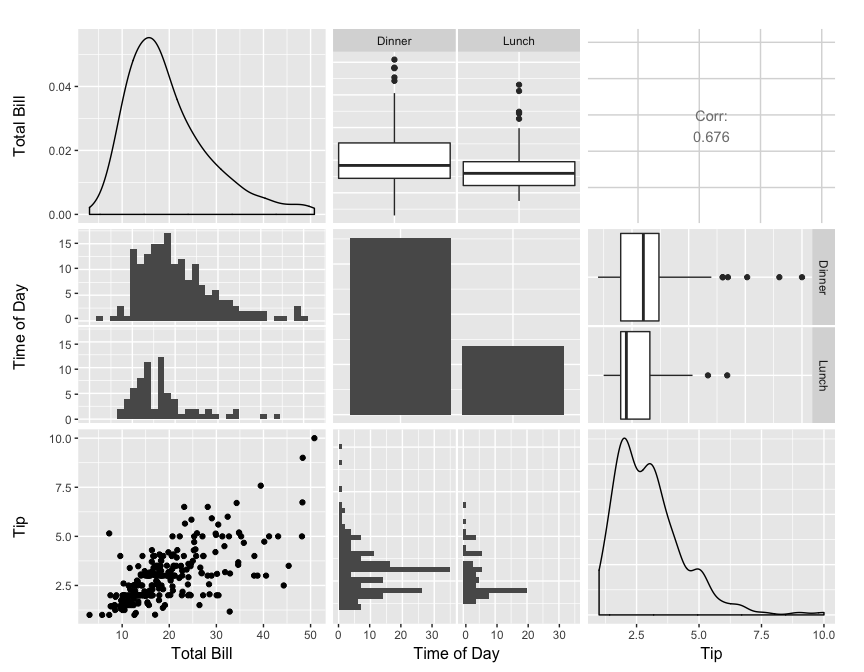# ggpairs

`ggpairs` is a special form of a `ggmatrix` that produces a pairwise comparison of multivariate data. By default, `ggpairs` provides two different comparisons of each pair of columns and displays either the density or count of the respective variable along the diagonal. With different parameter settings, the diagonal can be replaced with the axis values and variable labels.

There are many hidden features within ggpairs. Please take a look at the examples below to get the most out of ggpairs.

## Columns and Mapping

The `columns` displayed default to all columns of the provided `data`. To subset to only a few columns, use the `columns` parameter.

``````data(tips, package = "reshape")
pm <- ggpairs(tips)
pm``````
``````## `stat_bin()` using `bins = 30`. Pick better value with `binwidth`.
## `stat_bin()` using `bins = 30`. Pick better value with `binwidth`.
## `stat_bin()` using `bins = 30`. Pick better value with `binwidth`.
## `stat_bin()` using `bins = 30`. Pick better value with `binwidth`.
## `stat_bin()` using `bins = 30`. Pick better value with `binwidth`.
## `stat_bin()` using `bins = 30`. Pick better value with `binwidth`.
## `stat_bin()` using `bins = 30`. Pick better value with `binwidth`.
## `stat_bin()` using `bins = 30`. Pick better value with `binwidth`.
## `stat_bin()` using `bins = 30`. Pick better value with `binwidth`.
## `stat_bin()` using `bins = 30`. Pick better value with `binwidth`.
## `stat_bin()` using `bins = 30`. Pick better value with `binwidth`.
## `stat_bin()` using `bins = 30`. Pick better value with `binwidth`.````````````## too many plots for this example.

## reduce the columns being displayed
## these two lines of code produce the same plot matrix
pm <- ggpairs(tips, columns = c(1, 6, 2))
pm <- ggpairs(tips, columns = c("total_bill", "time", "tip"), columnLabels = c("Total Bill", "Time of Day", "Tip"))
pm``````
``````## `stat_bin()` using `bins = 30`. Pick better value with `binwidth`.
## `stat_bin()` using `bins = 30`. Pick better value with `binwidth`.``````Aesthetics can be applied to every subplot with the `mapping` parameter.

``````library(ggplot2)
pm <- ggpairs(tips, mapping = aes(color = sex), columns = c("total_bill", "time", "tip"))
pm``````
``````## `stat_bin()` using `bins = 30`. Pick better value with `binwidth`.
## `stat_bin()` using `bins = 30`. Pick better value with `binwidth`.``````